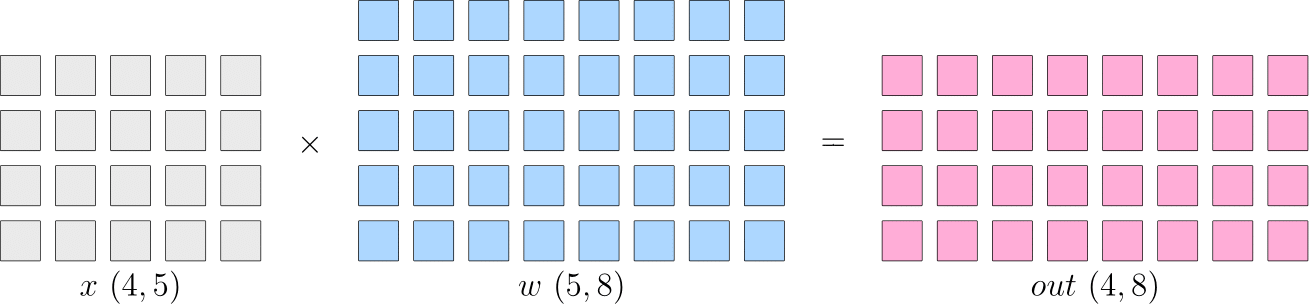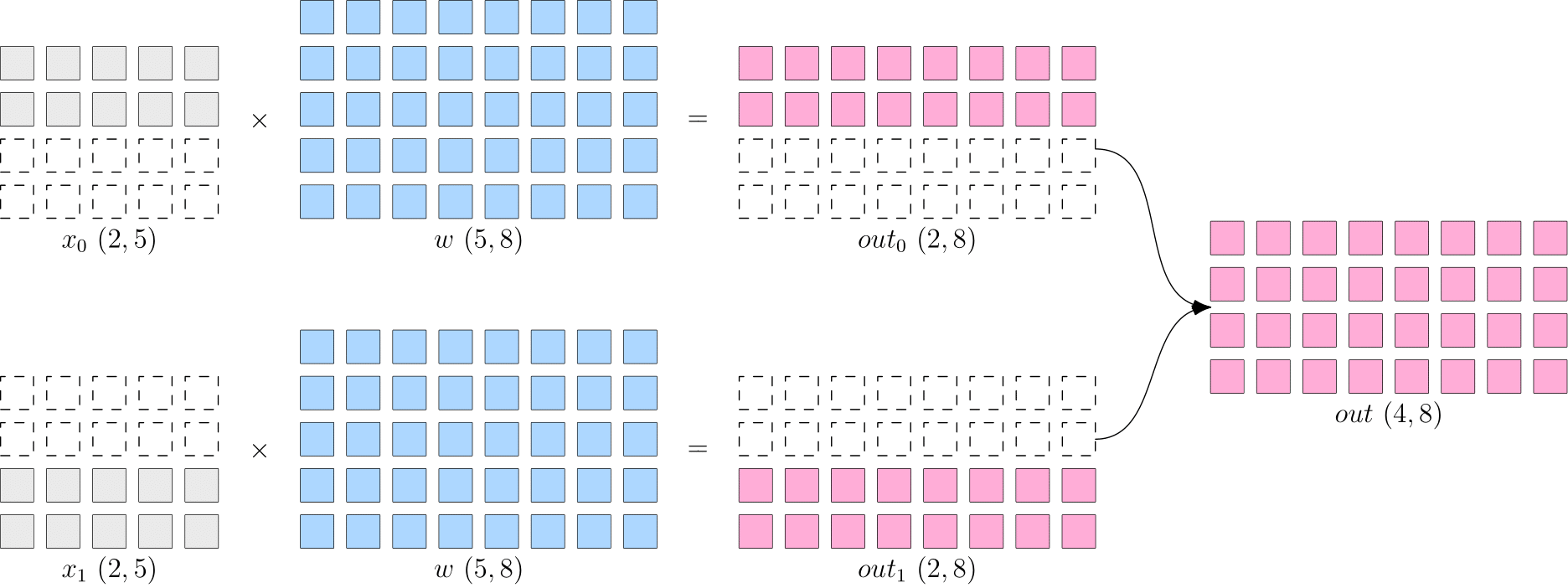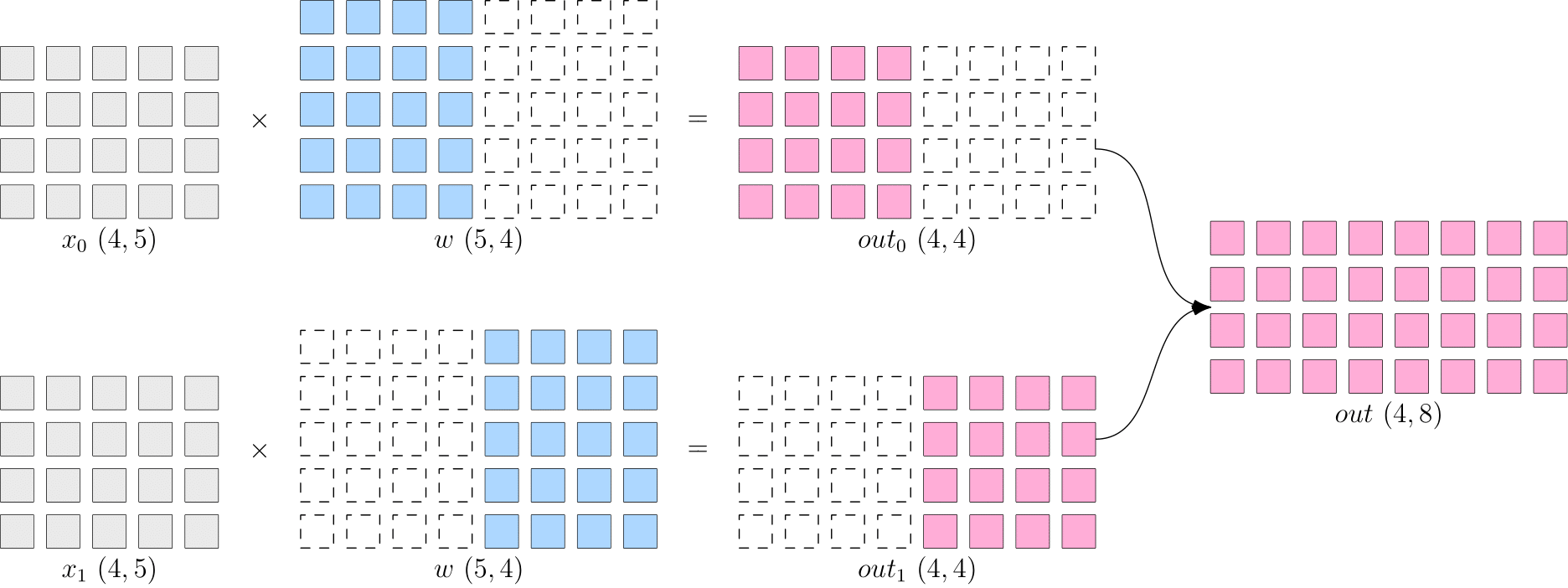# 使用 Global Tensor 进行分布式编程：分布式并行策略¶

## 并行策略¶

• 数据并行：对 数据 进行切分，不同设备数据不同，但模型相同
• 模型并行：对 模型 进行切分，不同设备数据相同，但模型不同
• 流水并行：将 模型 分为多个阶段，分发到不同设备，各个设备之间以“流水线”的方式完成训练

import oneflow as flow

x = flow.randn(4, 5)
w = flow.randn(5, 8)
out = flow.matmul(x, w)
print(out.shape) # (4, 8)### 数据并行¶

OneFlow 特有的 Global Tensor 采用 placementsbp 结合的方式完成分布。其中 placement 表示 Global Tensor 分布的物理设备，sbp 表示 Global Tensor 分布的方式（详情可见：创建 Global Tensor）。

import oneflow as flow
placement = flow.placement(type="cuda", ranks=[0, 1])
x = flow.randn(4, 5, placement=placement, sbp=flow.sbp.split(dim=0))
w = flow.randn(5, 8, placement=placement, sbp=flow.sbp.broadcast)
out = flow.matmul(x, w)
print(out.shape) # (4, 8)


python3 -m oneflow.distributed.launch --nproc_per_node 2 test.py1. 数据 $$x$$ 按第 0 维度切分(sbp=flow.sbp.split(dim=0))，分布在两卡设备上(placement=flow.placement(type="cuda", ranks=[0, 1]))
2. 模型 $$w$$ 保持完整(sbp=flow.sbp.broadcast)，分布在两卡设备上(placement=flow.placement(type="cuda", ranks=[0, 1]))

### 模型并行¶

import oneflow as flow

placement = flow.placement(type="cuda", ranks=[0, 1])
x = flow.randn(4, 5, placement=placement, sbp=flow.sbp.broadcast)
w = flow.randn(5, 8, placement=placement, sbp=flow.sbp.split(dim=1))
out = flow.matmul(x, w)
print(out.shape) # (4, 8)


python3 -m oneflow.distributed.launch --nproc_per_node 2 test.py1. 数据 $$x$$ 保持完整(sbp=flow.sbp.broadcast)，分布在两卡设备上(placement=flow.placement(type="cuda", ranks=[0, 1]))
2. 模型 $$w$$ 按第 1 维度切分(sbp=flow.sbp.split(dim=1))，分布在两卡设备上(placement=flow.placement(type="cuda", ranks=[0, 1]))

### 流水并行¶

import oneflow as flow

P0 = flow.placement(type="cuda", ranks=)
P1 = flow.placement(type="cuda", ranks=)

# 模型第一阶段分布在第 0 卡
w0 = flow.randn(5, 8, placement=P0, sbp=BROADCAST)
# 模型第二阶段分布在第 1 卡
w1 = flow.randn(8, 3, placement=P1, sbp=BROADCAST)

# 随机生成数据模拟输入，注意第一阶段的数据分布在第 0 卡
in_stage0 = flow.randn(4, 5, placement=P0, sbp=BROADCAST)
out_stage0 = flow.matmul(in_stage0, w0)
print(out_stage0.shape) # (4, 8)

# 利用 to_global 将第二阶段的数据分布在第 1 卡
out_stage1 = flow.matmul(in_stage1, w1)
print(out_stage1.shape) # (4, 3)


python3 -m oneflow.distributed.launch --nproc_per_node 2 test.py


Global Tensor 的设计，使得计算过程中，只需通过 to_global(...) 方法调整上一阶段的输出数据的分布策略，作为下一阶段的输入数据即可。

### 混合并行¶

OneFlow 同时支持 Eager 模式Graph 模式 两种模型运行方式，二者均可用于并行计算策略。

• Eager 模式 是 OneFlow 的默认模式，网络模型继承自 nn.Module 模块。
• Graph 模式 需要自定义继承自 nn.Graph 的类，并对 Eager 模式 的网络模型进行复用。

Note

import oneflow as flow
import oneflow.nn as nn

P01 = flow.placement(type="cuda", ranks=[0, 1])
P23 = flow.placement(type="cuda", ranks=[2, 3])

class StageModule(nn.Module):
def __init__(self, in_dims, out_dims, placement=None, sbp=None):
super().__init__()
self.w = nn.Parameter(
flow.randn(in_dims, out_dims, placement=placement, sbp=sbp)
)

def forward(self, x):
out = flow.matmul(x, self.w)
return out

class ModuleModel(nn.Module):
def __init__(self):
super().__init__()

# 模型第一阶段在第 0 和第 1 卡上进行数据并行计算
self.m_stage0 = StageModule(5, 8, placement=P01, sbp=flow.sbp.broadcast)

# 模型第二阶段在第 2 和第 3 卡上进行模型并行计算
self.m_stage1 = StageModule(8, 3, placement=P23, sbp=flow.sbp.split(dim=1))

def forward(self, x):
# 第一阶段，数据切分在第 0 和第 1 卡，用于数据并行
out_stage0 = self.m_stage0(x)

# 第二阶段需要将输入数据还原完整，并转移至第 2 和第 3 卡，用于模型并行
out_stage1 = self.m_stage1(in_stage1)

return out_stage0, out_stage1

if __name__ == "__main__":
model = ModuleModel()
# 需要将输入数据切分，用于数据并行
in_stage0 = flow.randn(4, 5, placement=P01, sbp=flow.sbp.split(dim=0))
out_stage0, out_stage1 = model(in_stage0)
print(out_stage0.shape, out_stage1.shape)  # (4, 8) (4, 3)

import oneflow as flow
import oneflow.nn as nn

P01 = flow.placement(type="cuda", ranks=[0, 1])
P23 = flow.placement(type="cuda", ranks=[2, 3])

class StageModule(nn.Module):
def __init__(self, in_dims, out_dims, placement=None, sbp=None):
super().__init__()
self.w = nn.Parameter(
flow.randn(in_dims, out_dims, placement=placement, sbp=sbp)
)

def forward(self, x):
out = flow.matmul(x, self.w)
return out

class ModuleModel(nn.Module):
def __init__(self):
super().__init__()

# 模型第一阶段在第 0 和第 1 卡上进行数据并行计算
self.m_stage0 = StageModule(5, 8, placement=P01, sbp=flow.sbp.broadcast)

# 模型第二阶段在第 2 和第 3 卡上进行模型并行计算
self.m_stage1 = StageModule(8, 3, placement=P23, sbp=flow.sbp.split(dim=1))

def forward(self, x):
# 第一阶段，数据切分在第 0 和第 1 卡，用于数据并行
out_stage0 = self.m_stage0(x)

# 第二阶段需要将输入数据还原完整，并转移至第 2 和第 3 卡，用于模型并行
out_stage1 = self.m_stage1(in_stage1)

return out_stage0, out_stage1

# Graph
class GraphModel(nn.Graph):
def __init__(self):
super().__init__()
self.model = ModuleModel()
self.model.m_stage0.to(nn.graph.GraphModule).set_stage(stage_id=0, placement=P01)
self.model.m_stage1.to(nn.graph.GraphModule).set_stage(stage_id=1, placement=P23)

def build(self, x):
return self.model(x)

if __name__ == "__main__":
graph = GraphModel()
# 需要将输入数据切分，用于数据并行
in_stage0 = flow.randn(4, 5, placement=P01, sbp=flow.sbp.split(dim=0))
out_stage0, out_stage1 = graph(in_stage0)
print(out_stage0.shape, out_stage1.shape)  # (4, 8) (4, 3)Eager 模式Graph 模式 的运行方式一致，假设脚本文件名为 test.py

1. 单机四卡启动方式为：

python3 -m oneflow.distributed.launch --nproc_per_node 4 test.py

2. oneflow 分布式工具支持多机多设备并行，以 2 机 2 卡 环境为例，启动方式如下：

在 第 0 号机器上运行：

python3 -m oneflow.distributed.launch \
--nnodes=2 \
--node_rank=0 \
--nproc_per_node=2 \
--master_addr="192.168.1.1" \ # 第 0 号机器的 IP
--master_port=7788 \
test.py


在 第 1 号机器上运行：

python3 -m oneflow.distributed.launch \
--nnodes=2 \
--node_rank=1 \
--nproc_per_node=2 \
--master_addr="192.168.1.1" \ # 第 0 号机器的 IP
--master_port=7788 \
test.py


注意要将 master_addr 设置为第 0 号机器的 IP